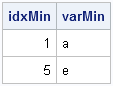The other day I encountered an article in the SAS Knowledge Base that shows how to write a macro that "returns the variable name that contains the maximum or minimum value across an observation." Some people might say that the macro is "clever." I say it is complicated. This is a simple problem; it deserves a simple solution.

This is one of those situations where a SAS/IML implementation is simpler and cleaner than a macro/DATA step solution. The following DATA step creates the data that are used in the SAS Knowledge Base article:

```/* Data for Sample 46471: Return the variable name that contains the max or min value across an observation */ data one; input a b c d e; cards; 1 3 12 6 15 34 583 294 493 2 ; run;```

By inspection, the minimum value in the first row is 1, which occurs for the variable A. In the second row, the minimum value is 2, which occurs for the variable E.

To find the variable for each row that contains the minimum value for that row, you can use the index minimum subscript reduction operator, which has the symbol >:<. The subscript reduction operators are a little-known part of the SAS/IML language, but they can be very useful. The following SAS/IML program begins by reading all numerical variables into a matrix, X. The subscript reduction operator then computes a column vector whose ith element is the column for which the ith row of X is minimal. You can use this column vector as an index into the names of the columns of X.

```/* For each row, find the variable name corresponding to the minimum value */ proc iml; use one; read all var _NUM_ into X[colname=VarNames]; close one;   idxMin = X[, >:<]; /* find columns for min of each row */ varMin = varNames[idxMin]; /* corresponding var names */ print idxMin varMin;```Yes, those two statements compute the same quantity as the complicated macro. And, if you are willing to nest the statements, you can combine them into a single statement:
varNames[X[, >:<]].

Finding the maximum value for each row is no more difficult: simply use the <:> subscript reduction operator.

The macro featured in the Knowledge Base article includes an option to compute with specified variables, rather than all numerical variables. That, too, is easily accomplished. For example, the following statements find the variable that has the largest value among the A, B, and C variables:

```varNames = {a b c}; use one; read all var varNames into X; close one;   idxMax = X[,<:>]; varMax = varNames[idxMax]; print idxMax varMax;```My next post will discuss subscript reduction operators in further details.

To my readers who are SQL experts: Is there a simple way to solve this problem by using PROC SQL? Leave a comment.